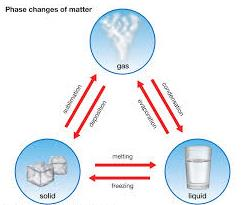Courses

# MCQ: Matter In Our Surroundings - 2

## 20 Questions MCQ Test Science Class 9 | MCQ: Matter In Our Surroundings - 2

Description
This mock test of MCQ: Matter In Our Surroundings - 2 for Class 9 helps you for every Class 9 entrance exam. This contains 20 Multiple Choice Questions for Class 9 MCQ: Matter In Our Surroundings - 2 (mcq) to study with solutions a complete question bank. The solved questions answers in this MCQ: Matter In Our Surroundings - 2 quiz give you a good mix of easy questions and tough questions. Class 9 students definitely take this MCQ: Matter In Our Surroundings - 2 exercise for a better result in the exam. You can find other MCQ: Matter In Our Surroundings - 2 extra questions, long questions & short questions for Class 9 on EduRev as well by searching above.
QUESTION: 1

### Which of the following statements is incorrect?

Solution:

Option A ,B and D all shows the property possessed by matter hence C is incorrect.

QUESTION: 2

### Which of the following is not a characteristic of matter?

Solution:

There is space between particles of matter in liquid and gases. Hence it is called intermolecular spaces .

option B is incorrect.

QUESTION: 3

### On converting 25°C, 38°C and 66°C to Kelvin scale, the correct sequence of temperature will be

Solution:

Kelvin is the SI Unit of measurement of temperatures. It is represented with the Unit Kelvin 'K'.

Temperatures can be converted from Celsius to Kelvin by adding 273 to the Celsius Scale.

We have been given 3 temperatures here which are in the Celsius Scale.

25°C, 38°C and 66°C

To convert all of them to the Kelvin Scale, add 273 to all of them.

25 + 273 = 298 K
38 + 273 = 311 K
66 + 273 = 339 K

QUESTION: 4

Shivam visited an LPG unit and found that the gas can be liquified at specific conditions of temperature and pressure. Help him to identify the correct set of conditions.

Solution:

High pressure and low temperature allows the particle to come closer that compresses a liquid to form gas.

QUESTION: 5

The property to flow is unique to fluids. Which one of the following statements is correct?

Solution:

Because liquid and gases have the tendency to flow.

QUESTION: 6

Which of the following is correct order of forces of attraction?

Solution:

solids have more force of attraction than liquids and gases.

QUESTION: 7

Which condition out of the following will increase the evaporation of water?

Solution:

Increase in temperature of water increases kinetic energy hence increases rate of evaporation.

QUESTION: 8

Choose the correct statement out of the following.

Solution:QUESTION: 9

Which one of the following sets of phenomenon would increase on raising the temperature?

Solution:

Evaporation ,diffusion and expansion of gases increase the temperature. Evaporation rate increases because of increasing temperature ,kinetic energy of molecules increases , so the molecules present at the surface of the liquid leave the surface quickly and go into the vapour state.

QUESTION: 10

Which of the following conditions will increase the rate of evaporation?

Solution:

In evaporation when the temperature is increased the molecules gain kinetic energy and molecules start to move faster and in order to become ga, it must overcome intermolecular forces between different molecules. Increasing the speed, molecules break free from bonds and evaporates.

QUESTION: 11

Seema visited a Natural Gas Compressing Unit and found that the gas can be liquefied under specific conditions of temperature and pressure. While sharing her experience with friends, she got confused. Help her to identify the correct set of conditions.

Solution:

Low temperature and high pressure are required to liquefy gases to liquids. There is a lot of space between the particles of a gas. On applying high pressure, the particles of gas move get so close that they start attracting each other sufficiently forming a liquid. When gas is compressed too much, heat is produced, so it is necessary to cool it. Cooling lowers the temperature of compressed gas and helps in liquefying it. Hence, a gas can be liquefied by applying high pressure and lowering the temperature (cooling).

QUESTION: 12

Which of the following is correct order of density?

Solution:

Solids have more density than liquids and gases.

QUESTION: 13

As the solid melts to form liquid,

Solution:

All three phenomena occurs when solid melts to form liquid hence option D is correct.

QUESTION: 14

The physical state of a matter depends upon

Solution:

Temperature and pressure both controls  the state of a matter.

QUESTION: 15

Which of the following does not affect the rate of evaporation?

Solution:

Insoluble impurities do not affect the rate of evaporation.

QUESTION: 16

Latent heat of fusion is amount of

Solution:

The heat  energy  required  to change  the state  of unit mass of  a substance  is called  latent  heat.  As the given solid is of unit mass  ( 1 kg)   and  we have  to change   its state into  liquid  , therefore  heat  needed will  be specific  latent heat of  fusion.

QUESTION: 17

Which of the following does not undergo sublimation?

Solution:

Sublimation is when a solid changes directly to gas without changing into liquid.
NaCl when heated does not change its state when heated , while all the other options sublime. Hence sodium chloride is the right answer.

QUESTION: 18

Which of the following is not correct?

Solution:

SI unit of pressure is Pascal

QUESTION: 19

Solids do not flow because

Solution:

Any thing flows due to weaker intermolecular forces between them.

QUESTION: 20

Which of the following can not be separated by sublimation?

Solution:

Sublimation is the transition of a substance directly from the solid to the gas phase without passing through the intermediate liquid phase. Ammonium chloride, Camphor, Anthracene, Naphthalene can sublime but common salt cannot sublime.